qtCircles
qtcircles Module Reference

# qtCircles

MODULE qtCircles provides procedures for calculating various quantities related to circles in 2-dimensional space (in the plane).
For documentation of the qtCircles module, the documentation tool "doxygen" has been used.
It lists symbols (names of functions, subroutines, parameters) being defined as PUBLIC in the module as members of the module. Therefore, you will find functions and subroutines being part of the "Data Types List". More...

## Public Member Functions

integer(kint) function, public qtcircles_initialize (cLicenceNumber)

# qtCircles_Initialize

Before using a qtCircles' function call qtCircles_Initialize for initialization. More...

real(kreal) function, public qtcircles_calc_y (x0, y0, R, X, iCtrl)

# qtCircles_Calc_Y

Calculates a single y-coordinate value (upper,lower,offset) of the circle specified by (x0,y0,R) for given x according to the circle's formula   $$R^2=(x-x_0)^2+(y-y_0)^2$$. More...

subroutine, public qtcircles_calc_yarr (x0, y0, R, N, aX, iCtrl, aY)

# qtCircles_Calc_YArr

Calculates y-coordinates (upper,lower,offset) for the circle specified by (x0,y0,R) for given list of x coordinates according to the circle's formula   $$R^2=(x-x_0)^2+(y-y_0)^2$$. More...

real(kreal) function, public qtcircles_calc_angle (x0, y0, R, X, iCtrl, Y)

# qtCircles_Calc_Angle

Calculates the angle between the x-axis and the radius vector from the circle's origin to a point on the circle. More...

real(kreal) function, public qtcircles_calc_dydx (x0, y0, R, X, iCtrl, Y)

# qtCircles_Calc_dYdX

Calculates the first derivative $$y'(x) = \frac{dy(x)}{dx}$$ at given coordinate x of the circle specified by (x0, y0, R). More...

real(kreal) function, public qtcircles_calc_d2ydx2 (x0, y0, R, X, iCtrl, Y)

# qtCircles_Calc_d2YdX2

Calculates the second derivative $$y''(x) = \frac{d^2y(x)}{dx^2}$$ at given coordinate x of the circle specified by (x0, y0, R). More...

integer(kint) function, public qtcircles_calc_params (aX, aY, x0, y0, R)

# qtCircles_Calc_Params

More...

integer(kint) function, public qtcircles_fit (N, aX, aY, x0, y0, R, Eps, MaxIt)

# qtCircles_Fit

More...

subroutine, public qtcircles_intersectioncircleline (x0, y0, r, a, b, nS, aXs, aYs)

# qtCircles_IntersectionCircleLine

Calculates the intersection points of a line intersecting a circle. More...

subroutine, public qtcircles_circlesintersection (x10, y10, r1, x20, y20, r2, nS, aXs, aYs)

# qtCircles_CirclesIntersection

Calculates the intersection points of two circles intersecting each other. More...

real(kreal) function, public qtcircles_circularsegmentarea (x0, y0, R, A, B, nS, aXs, aYs)

# qtCircles_CircularSegmentArea

A circle given by its origin (x0,y0) and its radius R is intersected by a secant. The "smaller" area between the circle and the secant - the segment - is calculated. More...

integer function, public qtcircles_tangentpoints (x0, y0, R, xP, yP, aXt, aYt, Eps)

real(kreal) function, public qtcircles_set_eps (Eps)

# qtCircles_Set_Eps

Sets the the internal threshold value for testing a value against 0:
x is meant to be 0, if |x| < ε
qtCircles_Set_Eps returns the previous threshold value. More...

## Public Attributes

integer, parameter, public kint = C_LONG
default KIND for INTEGER

integer, parameter, public kreal = C_DOUBLE
default KIND for REAL

real(kreal), parameter, public qtcircles_pi = 3.1415926535897932384626433832795D0
constant π

# qtCircles

MODULE qtCircles provides procedures for calculating various quantities related to circles in 2-dimensional space (in the plane).
For documentation of the qtCircles module, the documentation tool "doxygen" has been used.
It lists symbols (names of functions, subroutines, parameters) being defined as PUBLIC in the module as members of the module. Therefore, you will find functions and subroutines being part of the "Data Types List".

Usage
Specifiy the qtCircles MODULE in the program unit where you want to call a qtCircles procedure.

USE qtCircles


This also means, you need to specify the MODULE path of the file qtCircles.mod such that the compiler can find it.
And also, for correct linking, the qtCircles library (.lib) appropriate to your compiler has to be referenced (for example: for Intel Visual Fortran link qtCirclesIVF32.lib when creating a 32-bit application).

Initialization
Before calling any qtCircles' procedure, its functionality has to be initialized (one-time). Call qtCircles_Initialize for this purpose. For example:

 iRet = qtCircles_Initialize('L2890-123456')     ! if L2890-123456 is your qtCircles' licence number
x = SQRT(3)
dydx = qtCircles_Calc_dYdX( 0., 0.D0, 1.D0, x, 1, Y )


Internally, qtCircles_Initialize calls the licensing subroutine qtSetLicence_QTCIRCLES. If you have received a licence file (a file named "qtSetLicence_2890_123456.f90", for example) add this file to your programming project, such that it is compiled and linked.
If you run qtCircles in demonstration mode, compile the file qtSetLicence_QTCIRCLES.f90 and link the result (.obj).

Version
Release 1.00: 25. June 2014

## Member Function/Subroutine Documentation

 real(kreal) function, public qtcircles::qtcircles_calc_angle ( real(kreal), intent(in) x0, real(kreal), intent(in) y0, real(kreal), intent(in) R, real(kreal), intent(in) X, integer(kint), intent(in) iCtrl, real(kreal), intent(out), optional Y )

# qtCircles_Calc_Angle

Calculates the angle between the x-axis and the radius vector from the circle's origin to a point on the circle.

Usage:

Phi = qtCircles_Calc_Angle( x0, y0, R, X, iCtrl )


The input coordinates are cartesian. The angle is returned in radians.

Parameters
 x0,y0,R : origin (x0,y0) and radius R of the circle X : x-coordinate for which to calculate the angle iCtrl : value to control, which angle to calculate: =1: angle between positive x-axis and radius vector to point at the upper y-coordinate =2: angle between positive x-axis and radius vector to point at the lower y-coordinate =3: angle ("principal value") between x-axis and radius vector to point at the upper y-coordinate =4: angle ("principal value") between x-axis and radius vector to point at the lower y-coordinate Y : [optional] y-coordinate calculated for given x-coordinate

Usually for each x value two points on the circle exist and then at least two angles of the vector from the circle's origin to the point on the circle ("the radius vector") can be measured against the x-axis. Which of these to be calculated is controlled by dummy argument iCtrl.
If iCtrl = 1, the angle of the radius vector to the point of the upper y-coordinate (y ≥ y0) is measured counter-clockwise against the positive x-axis (see φ1 in illustration below).
If iCtrl = 2, the angle of the radius vector to the point of the lower y-coordinate (y ≤ y0) is measured counter-clockwise against the positive x-axis (see φ2 in illustration below).
If iCtrl = 3, the angle of the radius vector to the point of the upper y-coordinate (y ≥ y0) is measured against the x-axis (see φ3 in illustration below). This gives the ArcTan of $$\frac{y - y0}{x - x0}$$.
If iCtrl = 4, the angle of the radius vector to the point of the lower y-coordinate (y ≤ y0) is measured against the x-axis (see φ4 in illustration below). This gives the ArcTan of $$\frac{y - y0}{x - x0}$$.
If x is outside of the circle's definition (x < x0 - R or x > x0 + R), the function returns the value of HUGE(Phi).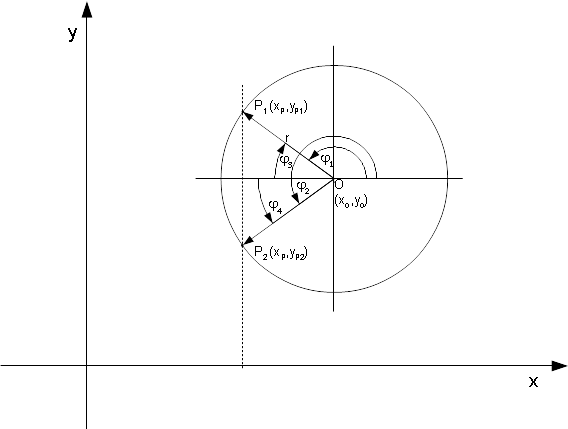real(kreal) function, public qtcircles::qtcircles_calc_d2ydx2 ( real(kreal), intent(in) x0, real(kreal), intent(in) y0, real(kreal), intent(in) R, real(kreal), intent(in) X, integer(kint), intent(in) iCtrl, real(kreal), intent(out), optional Y )

# qtCircles_Calc_d2YdX2

Calculates the second derivative $$y''(x) = \frac{d^2y(x)}{dx^2}$$ at given coordinate x of the circle specified by (x0, y0, R).

Usage:

d2YdX2 = qtCircles_Calc_d2YdX2( x0, y0, R, X, iCtrl )
d2YdX2 = qtCircles_Calc_d2YdX2( x0, y0, R, X, iCtrl, Y )


All coordinates are cartesian.

Usually for each x value two values for the derivative $$y''(x) = \frac{d^2y(x)}{dx^2}$$ exist, so you have to specify which one you want.

Parameters
 x0,y0,R : origin (x0,y0) and radius R of the circle X : x-coordinate for which to calculate the derivative iCtrl : value to control, whether the derivative at the upper (=1) or the lower (=-1) y(x)-coordinate shall be calculated Y : [optional] y-coordinate calculated for given x-coordinate

Depending on the value of argument iCtrl, the function returns

 d2ydx2U = qtCircles_Calc_d2YdX2( x0, y0, R, x, 1, yU )   ! at upper y value
d2ydx2L = qtCircles_Calc_d2YdX2( x0, y0, R, x, -1 )      ! at lower y value


If x = x0 + R, y becomes y0, and the derivative becomes infinite. Then the function returns the value of -HUGE(X).
If x = x0 - R, y becomes y0, and the derivative becomes infinite. Then the function returns the value of HUGE(X).
If x is outside of the circle's definition (x < x0 - R or x > x0 + R), the function returns the value of HUGE(Y) if x < x0, otherwise -HUGE(Y) is returned.

 real(kreal) function, public qtcircles::qtcircles_calc_dydx ( real(kreal), intent(in) x0, real(kreal), intent(in) y0, real(kreal), intent(in) R, real(kreal), intent(in) X, integer(kint), intent(in) iCtrl, real(kreal), intent(out), optional Y )

# qtCircles_Calc_dYdX

Calculates the first derivative $$y'(x) = \frac{dy(x)}{dx}$$ at given coordinate x of the circle specified by (x0, y0, R).

Usage:

dydx = qtCircles_Calc_dYdX( x0, y0, R, X, iCtrl )
dydx = qtCircles_Calc_dYdX( x0, y0, R, X, iCtrl, Y )


All coordinates are cartesian.

Usually for each x value two values for the derivative $$\frac{dy(x)}{dx}$$ exist, so you have to specify which one you want.

Parameters
 x0,y0,R : origin (x0,y0) and radius R of the circle X : x-coordinate for which to calculate the derivative iCtrl : value to control, whether the derivative at the upper (=1) or the lower (=-1) y(x)-coordinate shall be calculated Y : [optional] y-coordinate calculated for given x-coordinate

Depending on the value of argument iCtrl, the function returns

 dydxU = qtCircles_Calc_dYdX( x0, y0, R, x, 1 )       ! at upper y value
dydxL = qtCircles_Calc_dYdX( x0, y0, R, x, -1, yL )  ! at lower y value


If x = x0 + R, y becomes y0, and the derivative becomes infinite. Then the function returns the value of -HUGE(X).
If x = x0 - R, y becomes y0, and the derivative becomes infinite. Then the function returns the value of HUGE(X).
If x is outside of the circle's definition (x < x0 - R or x > x0 + R), the function returns the value of HUGE(Y) if x < x0, otherwise -HUGE(Y) is returned.

 integer(kint) function, public qtcircles::qtcircles_calc_params ( real(kreal), dimension(n), intent(in) aX, real(kreal), dimension(n), intent(in) aY, real(kreal), intent(inout) x0, real(kreal), intent(inout) y0, real(kreal), intent(inout) R )

# qtCircles_Calc_Params

Calculates the circle's parameters x0, y0 and R for three given points according to the circle's formula   $$R^2=(x-x_0)^2+(y-y_0)^2$$.

Usage:

iRet = qtCircles_Calc_Params( aX, aY, x0, y0, R )


All coordinates are cartesian.

Parameters
 aX(:) : array containing x-coordinates of three points that determine the circle; dimension: ≥ 3 aY(:) : array containing y-coordinates of three points that determine the circle; dimension: ≥ 3 x0,y0,R : on return, the origin (x0,y0) and the radius R of the circle

If a solution could be found, then the function returns the value 1.
If points are identical, the function returns a negative value (either -1 or -2).

 real(kreal) function, public qtcircles::qtcircles_calc_y ( real(kreal), intent(in) x0, real(kreal), intent(in) y0, real(kreal), intent(in) R, real(kreal), intent(in) X, integer(kint), intent(in) iCtrl )

# qtCircles_Calc_Y

Calculates a single y-coordinate value (upper,lower,offset) of the circle specified by (x0,y0,R) for given x according to the circle's formula   $$R^2=(x-x_0)^2+(y-y_0)^2$$.

Usage:

y = qtCircles_Calc_Y( x0, y0, R, x, iCtrl )


All coordinates are cartesian.

Usually for each x value two y-coordinates exist, so you have to specify which one you want.

Parameters
 x0,y0,R : origin (x0,y0) and radius R of the circle X : x-coordinate for which to calculate the y-coordinate iCtrl : value to control, whether the upper (=1), lower (=-1) or the y0 offset (=0) for y shall be calculated

Depending on the value of argument iCtrl, the function returns

 y = y0 + qtCircles_Calc_Y( x0, y0, R, x, 1 )   ! upper y value
y = y0 + qtCircles_Calc_Y( x0, y0, R, x, -1 )  ! lower y value
yOffset = qtCircles_Calc_Y( x0, y0, R, x, 0 )  ! offset to be added to y0 or substracted from y0
yU = y0 + yOffset   ! upper y value
yL = y0 - yOffset  ! lower y value


If x is outside of the circles definition (x < x0 - R or x > x0 + R), the function returns the value of HUGE(Y).

Annotation: To calculate a single y-coordinate use qtCircles::qtcircles_calc_yarr.

 subroutine, public qtcircles::qtcircles_calc_yarr ( real(kreal), intent(in) x0, real(kreal), intent(in) y0, real(kreal), intent(in) R, integer(kint), intent(in) N, real(kreal), dimension(n), intent(in) aX, integer(kint), intent(in) iCtrl, real(kreal), dimension(n), intent(out) aY )

# qtCircles_Calc_YArr

Calculates y-coordinates (upper,lower,offset) for the circle specified by (x0,y0,R) for given list of x coordinates according to the circle's formula   $$R^2=(x-x_0)^2+(y-y_0)^2$$.

Usage:

 CALL qtCircles_Calc_YArr( x0, y0, R, N, aX, iCtrl, aY )


All coordinates are cartesian.

Usually for each x value two y-coordinates exist, so you have to specify which one you want.

Parameters
 x0,y0,R : origin (x0,y0) and radius R of the circle N : number of x-values in aX(:) and minimal dimension of arrays aX(:) and aY(:) aX(:) : array containing x-coordinates for which to calculate the y-coordinates; required dimension of aX(M): M ≥ N iCtrl : value to control, whether the upper (=1), lower (=-1) or the y0 offset (=0) for y shall be calculated aY(:) : array of y-coordinates being calculated on return; required dimension of aY(M): M ≥ N
 CALL qtCircles_Calc_YArr( x0, y0, R, N, aX, 1, aY )    ! upper y values
CALL qtCircles_Calc_YArr( x0, y0, R, N, aX, -1, aY )   ! lower y values
CALL qtCircles_Calc_YArr( x0, y0, R, N, aX, 0, aY )    ! offsets to be added to y0 or substracted from y0
aY(:) = y0 - aY(:)   ! lower y values, for example


If aX(j) is outside of the circles definition ( aX(j) < x0 - R or aX(j) > x0 + R ), the routine returns aY(j) = HUGE(aY).

Annotation: To calculate a single y-coordinate use qtCircles::qtcircles_calc_y.

 subroutine, public qtcircles::qtcircles_circlesintersection ( real(kreal), intent(in) x10, real(kreal), intent(in) y10, real(kreal), intent(in) r1, real(kreal), intent(in) x20, real(kreal), intent(in) y20, real(kreal), intent(in) r2, integer(kint), intent(out) nS, real(kreal), dimension(2), intent(out) aXs, real(kreal), dimension(2), intent(out) aYs )

# qtCircles_CirclesIntersection

Calculates the intersection points of two circles intersecting each other.

Usage:

CALL qtCircles_CirclesIntersection( x10, y10, r1, x20, y20, r2, nS, aXs, aYs )


All coordinates are cartesian.

Parameters
 x10,y10,r1 : origin (x10,y10) and radius r1 of the first circle x20,y20,r2 : origin (x20,y20) and radius r2 of the second circle nS : on return, the number of the intersection points found nS=2: the circles intersect in two points nS=1: the circles touch each other nS=0: the circles neither intersect nor touch each other nS=HUGE(nS): the circles are identical aXs,aYs : on return, the coordinates of the intersection points if nS=2, or if nS=1 the coordinates of the point where the circles touch. Otherwise: aXs(:) and aYs(:) left unchanged. Dimension: 2

The procedure calculates the intersection points of two circles intersecting each other (nS returns 2), unless both circles just touch (nS returns 1) or they don't intersect or touch themselves at all (nS returns 0).
Both circles are given by their parameters xj0, yj0, and rj ( $$r_j^2=(x-x_{j0})^2+(y-y_{j0})^2$$) with j = 1, 2.
If the circles just touch, then the coordinates returned in the dummy arrays are identical (i.e.: aXs(2)=aXs(1) and aYs(2)=aYs(1)).
If the circles are identical, the dummy argument nS returns HUGE(nS).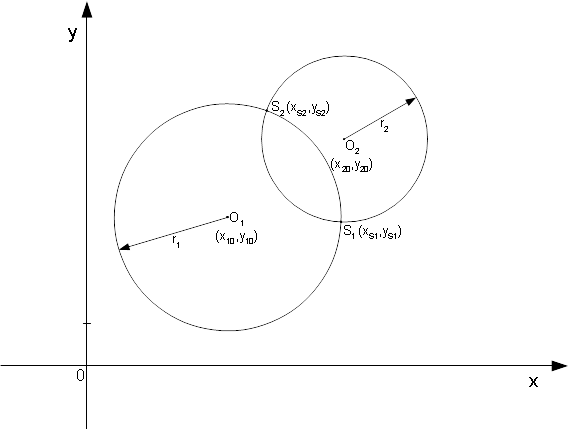real(kreal) function, public qtcircles::qtcircles_circularsegmentarea ( real(kreal), intent(in) x0, real(kreal), intent(in) y0, real(kreal), intent(in) R, real(kreal), intent(in) A, real(kreal), intent(in) B, integer(kint), intent(out), optional nS, real(kreal), dimension(2), intent(out), optional aXs, real(kreal), dimension(2), intent(out), optional aYs )

# qtCircles_CircularSegmentArea

A circle given by its origin (x0,y0) and its radius R is intersected by a secant. The "smaller" area between the circle and the secant - the segment - is calculated.

Usage:

CSA = qtCircles_CircularSegmentArea( x0, y0, R, A, B )
CSA = qtCircles_CircularSegmentArea( x0, y0, R, A, B, aXs, aYs )


All coordinates are cartesian.

Parameters
 x0,y0,R : origin (x10,y10) and radius r1 of the first circle a,b : parameters describing the secant according to the line's formula $$y(x) = a x + b$$ nS : [optional] on return, the number of intersection points found nS=2: the line intersects the circle in two points nS=1: the line is a tangent to the circle nS=0: the line does not intersect the circle (not a secant) aXs(:),aYs(:) : [optional] on return, the coordinates of the intersection points; dimension: 2

A circle given by its origin (x0,y0) and its radius R according to $$R^2=(x-x_0)^2+(y-y_0)^2$$) is intersected by a line with parameters (A, B) according to $$y(x) = A x + B$$, the secant. The "smaller" area between the circle and the secant - the segment - is calculated.
If the line is a tangent to the circle or it doesn't even intersect the circle, the function returns the value 0.
If the line is a secant and dummy arrays aXs(:) and aYs(:) are specified, on return of the function these arrays contain the coordinates of the intersection points.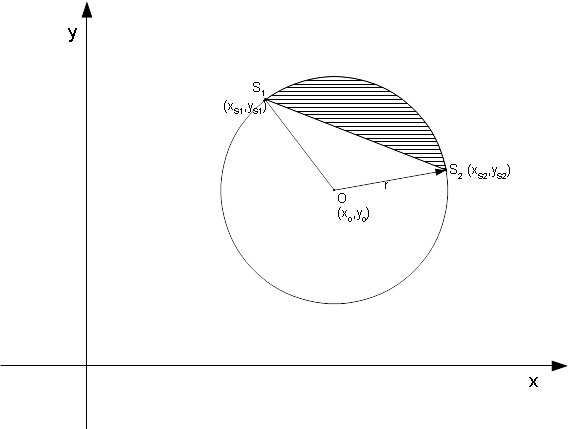integer(kint) function, public qtcircles::qtcircles_fit ( integer(kint), intent(in) N, real(kreal), dimension(n), intent(in) aX, real(kreal), dimension(n), intent(in) aY, real(kreal), intent(inout) x0, real(kreal), intent(inout) y0, real(kreal), intent(inout) R, real(kreal), intent(in), optional Eps, integer(kint), intent(inout), optional MaxIt )

# qtCircles_Fit

Determines a circle's parameters x0, y0, and R for three or more given points lying on or near to the circle specified by   $$R^2=(x-x_0)^2+(y-y_0)^2$$.   The parameters are fitted using the least square method.

Usage:

iRet = qtCircles_Fit( N, aX, aY, x0, y0, R )
iRet = qtCircles_Fit( N, aX, aY, x0, y0, R, Eps )
iRet = qtCircles_Fit( N, aX, aY, x0, y0, R, Eps, MaxIt )


All coordinates are cartesian.

Parameters
 N : number of points points to determine the circle aX(:) : array containing x-coordinates of points that determine the circle; dimension: N ≥ 3 aY(:) : array containing y-coordinates of points that determine the circle; dimension: N ≥ 3 x0,y0,R : on entry: initialization values for x0, y0, and R, respectively on return: the origin (x0,y0) and the radius R of the circle fitted to the given points Eps : [optional] threshold value to check the approximation; should be close to 0, for example 0.00001. MaxIt : [optional]

The function tries to determine the circle's parameters x0, y0, and R by using the least square method in an iterative process. The maximum number of iterations is limited internally (1000 iterations). But this can be changed by specifying a value in dummy argument MaxIt.
The accuracy is determined by an internal threshold value of Eps, which is used to check if the change of the approximated parameters (x0, y0, R) in an interation loop is less or equal to this Eps value (i.e.: $$|r_{j+1} - r_j|$$ ≤ ε). If this is the case, the function returns the value 1 and a solution for x0, y0, and R.
The value for Eps can be changed by specifying a value in the dummy argument. If an Eps is specified, make sure that this is at least greater than 10*EPSILON(0.D0), otherwise the iteration limit is easily reached. The default value for Eps is 0.00001 unless it has been changed by calling qtCircles_Set_Eps.
If only three points are given (N = 3), then qtCircles_Calc_Params is internally used to determine the circle's parameters. Then, a dummy argument MaxIt would return 0.
If no solution was found, the function returns a negative value with following meanings:
-1: N < 3 (at least 3 points are needed)
-2: R ≤ 0 on entry.
-3: the internal iteration limit was reached (increasing MaxIt or setting a bigger threshold Eps might help to obtain a solution).

 integer(kint) function, public qtcircles::qtcircles_initialize ( character (*), intent(in) cLicenceNumber )

# qtCircles_Initialize

Before using a qtCircles' function call qtCircles_Initialize for initialization.

Usage:

iRet = qtCircles_Initialize( cLicenceNumber )

Parameters
 cLicenceNumber : either a valid licence number (format: 'L####-######', with # being a digit) or the word 'evaluation', for which qtCircles will run in demo mode.

If a valid licence number or 'evaluation' was specified on entry, the function returns 0. Any other value returned indicates an error, and then qtCircles' functions will not work properly. qtCircles_Initialize has to be called only one-time per program run.

 subroutine, public qtcircles::qtcircles_intersectioncircleline ( real(kreal), intent(in) x0, real(kreal), intent(in) y0, real(kreal), intent(in) r, real(kreal), intent(in) a, real(kreal), intent(in) b, integer(kint), intent(out) nS, real(kreal), dimension(2), intent(out) aXs, real(kreal), dimension(2), intent(out) aYs )

# qtCircles_IntersectionCircleLine

Calculates the intersection points of a line intersecting a circle.

Usage:

CALL qtCircles_IntersectionCircleLine( x0, y0, r, a, b, nS, aXs, aYs )


All coordinates are cartesian.

Parameters
 x0,y0,R : origin (x0,y0) and radius r of the circle a,b : parameters describing the line according to $$y(x) = a x + b$$ nS : on return, the number of the coordinates found nS=2: the line intersects the circle in two points nS=1: the line is a tangent to the circle nS=0: the line does not intersect the circle aXs(:),aYs(:) : on return, the coordinates of the points that intersect the circle if nS=2, or if nS=1 the coordinates of the point where the line touches the circle; dimension: 2

The procedure calculates the two intersection points of a line intersecting a circle (nS returns 2), unless the line is a tangent to the circle (nS returns 1) or it doesn't intersect or touch the circle at all (nS returns 0).
The line is given by its parameters a and b ( $$y = a x + b$$ ) and the circle is given by its parameters x0, y0, and r ( $$r^2=(x-x_0)^2+(y-y_0)^2$$ ).
To specify a vertical line (x = const.), set a = HUGE(a) and set b equal to the x-coordinate (i.e.: x = b).
If the line is a tangent to the circle, then the coordinates returned in the dummy arrays are set identical (aXs(2)=aXs(1) and aYs(2)=aYs(1)).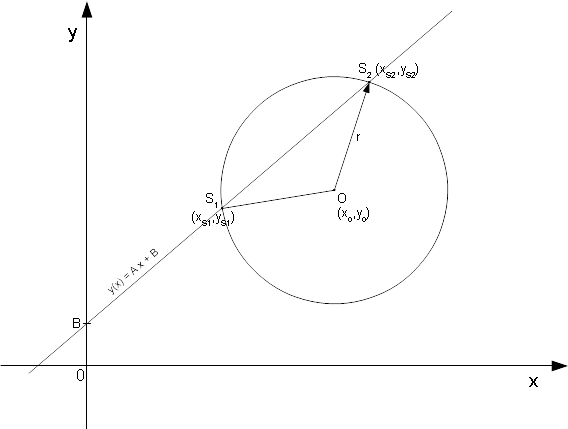real(kreal) function, public qtcircles::qtcircles_set_eps ( real(kreal), intent(in) Eps )

# qtCircles_Set_Eps

Sets the the internal threshold value for testing a value against 0:
x is meant to be 0, if |x| < ε
qtCircles_Set_Eps returns the previous threshold value.

Usage:

oldEps = qtCircles_Set_Eps( Eps )

Parameters
 Eps : threshold value, should be close to 0, for example 0.00001.
 integer function, public qtcircles::qtcircles_tangentpoints ( real(kreal), intent(in) x0, real(kreal), intent(in) y0, real(kreal), intent(in) R, real(kreal), intent(in) xP, real(kreal), intent(in) yP, real(kreal), dimension(2), intent(out) aXt, real(kreal), dimension(2), intent(out) aYt, real(kreal), intent(in), optional Eps )

# qtCircles_TangentPoints

Calculates the coordinates of the tangent points for the two lines from point P (xP, yP) that touch a circle defined by (x0,y0,R).

Usage:

nTp = qtCircles_TangentPoints( x0, y0, R, xP, yP, aXt, aYt[, Eps] )


All coordinates are cartesian.

Parameters
 x0,y0,R : origin (x0, y0) and radius R of the circle xP,yP : coordinates of point P aXt(:),aYt(:) : arrays of coordinates of tangent point(s) (if nTp > 0); dimension: ≥2 Eps : [optional] threshold for detecting if point P lies on the circle; default is 0.00001 (can be changed by qtCircles_Set_Eps)

If the function returns 2, there are two tangent points returned in aXt(1:2) and aYt(1:2).
If the function returns 1, then there is the single tangent point which is identical to P. I.e.: point P is on the circle). aXt(1) = aXt(2) = xP, and aYt(1) = aYt(2) = yP)
If the function returns 0, point P is within the circle and so, there are no tangent points. aXt(:) and aYt(:) are left unchanged.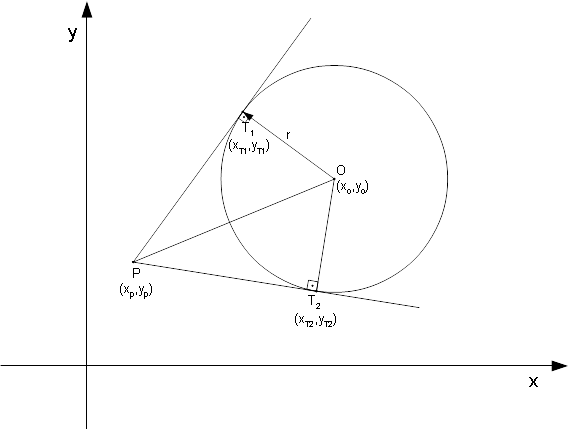Example

 USE qtCircles
REAL(KREAL) :: x0 = 2., y0 = 0., R = 1.
INTEGER nTp
REAL(KREAL) :: xP = 0., yP = 0.
REAL(KREAL) :: aXt(2), aYt(2)
nTp = qtCircles_TangentPoints( x0, y0, R, xP, yP, aXt, aYt )
! result:  nTp = 2, aXt(:) = (/1.5, 1.5/), aYt(:) = (/0.866025, -0.866025/)